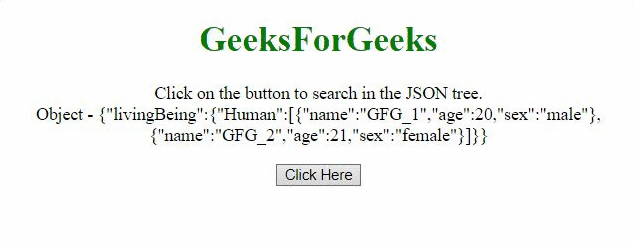Open in App
Not now

# How to search JSON tree with the help of jQuery ?

• Last Updated : 24 Jan, 2023

In this article, we are given a JSON Object’s tree and the task is to find a particular piece of information. Suppose we want to find the information about a particular person in that object then how do we do that? Here are two approaches that are discussed below:

Approach 1: In this approach, we will use each() method and pass the real array of objects to the method by using the dot operator, and then in the anonymous function find the person by comparing its name.

Example: This example shows the use of the above-explained approach.

## HTML

 `<``head``> ` `    ``<``script` `src``= ` `"https://code.jquery.com/jquery-3.5.0.js"``> ` `    `` ` ` ` `<``body` `style``=``"text-align:center;"``> ` ` `  `    ``<``h1` `style``=``"color: green"``> ` `        ``GeeksForGeeks ` `    `` ` ` `  `    ``<``p` `id``=``"GFG_UP"``> ` ` `  `    ``<``button` `onclick``=``"gfg_Run()"``> ` `        ``Click Here ` `    `` ` ` `  `    ``<``p` `id``=``"GFG_DOWN"``> ` ` `  `    ``<``script``> ` `        ``var elUp = document.getElementById("GFG_UP"); ` `        ``var elDown = document.getElementById("GFG_DOWN"); ` `         `  `        ``var GFG_Object = { ` `            ``"livingBeing": { ` `                ``"Human": [ ` `                    ``{ ` `                        ``"name": "GFG_1", ` `                        ``"age": 20, ` `                        ``"sex": "male" ` `                    ``}, { ` `                        ``"name": "GFG_2", ` `                        ``"age": 21, ` `                        ``"sex": "female" ` `                    ``} ` `                ``] ` `            ``} ` `        ``}; ` `         `  `        ``elUp.innerHTML = ` `            ``"Click on the button to search in" ` `            ``+ " the JSON tree.<``br``>Object - " ` `            ``+ JSON.stringify(GFG_Object); ` `         `  `        ``function gfg_Run() { ` `            ``\$.each(GFG_Object.livingBeing.Human,  ` `                ``function (i, v) { ` `                 `  `                ``if (v.name == "GFG_1") { ` `                    ``elDown.innerHTML =  ` `                        ``"The age of person('GFG_1') is  " ` `                        ``+ v.age; ` `                    ``return; ` `                ``} ` `            ``}); ` `        ``}    ` `    `` ` ``

Output:Approach 2: In this approach, we will use grep() method and pass the actual array of objects to the method by using the dot operator, then return the object which matches the criteria and then print the age of the person.

Example: This example shows the use of the above-explained approach.

## HTML

 `<``head``> ` `    ``<``script` `src``= ` `"https://code.jquery.com/jquery-3.5.0.js"``> ` `    `` ` ` ` `<``body` `style``=``"text-align:center;"``> ` ` `  `    ``<``h1` `style``=``"color: green"``> ` `        ``GeeksForGeeks ` `    `` ` ` `  `    ``<``p` `id``=``"GFG_UP"``> ` ` `  `    ``<``button` `onclick``=``"gfg_Run()"``> ` `        ``Click Here ` `    `` ` ` `  `    ``<``p` `id``=``"GFG_DOWN"``> ` ` `  `    ``<``script``> ` `        ``var elUp = document.getElementById("GFG_UP"); ` `        ``var elDown = document.getElementById("GFG_DOWN"); ` `        ``var GFG_Object = { ` `            ``"livingBeing": { ` `                ``"Human": [ ` `                    ``{ ` `                        ``"name": "GFG_1", ` `                        ``"age": 20, ` `                        ``"sex": "male" ` `                    ``}, { ` `                        ``"name": "GFG_2", ` `                        ``"age": 21, ` `                        ``"sex": "female" ` `                    ``} ` `                ``] ` `            ``} ` `        ``}; ` `        ``elUp.innerHTML ` `            ``= "Click on the button to search in" ` `            ``+ " the JSON tree.<``br``>Object - " ` `            ``+ JSON.stringify(GFG_Object); ` `         `  `        ``function gfg_Run() { ` `            ``var res ` `                ``= \$.grep(GFG_Object.livingBeing.Human,  ` `                ``function (element, index) { ` `                    ``return (element.name === 'GFG_1'); ` `                ``}); ` `            ``elDown.innerHTML =  ` `                ``"The age of person('GFG_1') is "  ` `                ``+ res.age; ` `        ``}    ` `    `` ` ``

Output:My Personal Notes arrow_drop_up
Related Articles